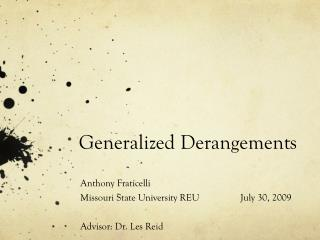# Generalized Derangements - PowerPoint PPT PresentationDownload PresentationGeneralized Derangements

Generalized Derangements
Download Presentation## Generalized Derangements

- - - - - - - - - - - - - - - - - - - - - - - - - - - E N D - - - - - - - - - - - - - - - - - - - - - - - - - - -
##### Presentation Transcript

1. Generalized Derangements Anthony Fraticelli Missouri State University REU July 30, 2009 Advisor: Dr. Les Reid

2. Overview • In this presentation we will address the following: • Introductory definitions • Classic derangements • k-derangements • Exponential generating functions • Limits • Rencontres numbers • k-rencontres numbers

3. Introductory Definitions • Permutation – A mapping of elements from a set to elements of the same set. Ex. • Cycle – A permutation of the elements of some set X which maps the elements of some subset S to each other in a cyclic fashion, while fixing all other elements. • Partition – A set of nonempty subsets of X such that every element x in X is in exactly one of these subsets.

4. What Are Classic Derangements? • The classic derangement problem asks, "How many permutations of n objects leave no elements fixed?" • For example, “If six students in a class all take a test, how many ways can the teacher pass back their exams so that none of the students receive their own test?” • The solution to this problem can be found by using one of the well known classic derangement formulas. • Using (1) it is clear to see that D6= 265. • From here we can compute the probability that a permutation is a derangement by dividing by the total number of possible permutations, n! Therefore, the probability that no student receives their own test in our example is D6/6! ≈ .368 • It is also well known that:

5. What Are k-Derangements? • Definition: • In other words, a permutation is a k -derangement if its cycle decomposition does not have any cycles whose lengths partition k. • For example, P{2,2} is a 3-derangement in S4 since neither of the cycles of length 2 can partition k=3. However, P{2,1,1} is not a 3-derangement since either combination of the cycles of length {2,1} partition k=3. • Being able to calculate k-derangements will allow us to ask questions such as, “How many ways can a teacher pass back a test such that no two students receive their own test or each others tests?”

6. Calculating k-Derangements • To calculate the number ofk-derangements by hand: • Start with the number of all permutations - n! • Divide by the length of each partition. • Distinguish between repeated partitions by dividing by r! where r is the number of times each partition is repeated. • Repeat this process and sum over all acceptable permutations of Pr. • Ex. D(2,6) P{6} P{5,1} P{3,3}

7. Table of k-Derangements

8. Exponential Generating Functions • A generating function is a formal power series whose coefficients encode information about a sequence an. • Exponential generating functions are represented as: • Proposition:Let Cl represent cycles of length l. Then the exponential generating function for Cl is the power series, centered at 0, whose coefficients of xn represent n numbers of Cl.

9. and • Since permutations can be represented as a product of disjoint cycles, the exponential generating function for all permutations is simply the multiplication of the EG’s for all of the cycles. • Also, since classic derangements cannot contain permutations whose cycle decompositions contain cycles of length 1, we can remove the EG for 1-cycles to obtain the EG for classic derangements.

10. EG’s for k-Derangements • Just as we found the EG for classic derangements, we can find the EG’s for k-derangements by removing from the EG of all permutations those cycles whose lengths partition k. • We have found the EG’s for k-derangements up to k=8, however we have not yet been able to generalize a pattern from the EG’s since they become increasingly complex.

11. Formulas for 2-Derangements • By performing the series expansion on it can be shown that: • D(2,n) also satisfies the following recurrence:

12. Limits of EG’s • Lemma • Proof

13. Limits of D(k,n)/n! • Using the previous lemma, we can find the limits of the first few values of D(k,n)/n! as n goes to infinity. • Conjecture –

14. Rencontres Numbers • Definition -For n ≥ 0 and 0 ≤ r ≤ n, the rencontres number D(r,n) is the number of permutations of [n] that have exactly r fixed points. • In other words, rencontres numbers specify how many permutations leave r number of 1-tuples fixed. • Note D(0,n) are classic derangements. • Known formulas for rencontres numbers include:

15. k-Rencontres Numbers • Definition -For n ≥ 0 ,0 ≤ r ≤ n/k, and , 0 ≤ k ≤ nk-rencontres numbers D(r,k,n) are the number of permutations that leave exactly r number of k-tuples fixed. • Note D(0,k,n) are k-derangements. • We have found EG’s for the following:

16. Unsolved Problems • Prove • Prove • Find a recursive formula, explicit formula, or exponential generating function for all k-derangements. • Find a recursive formula, explicit formula, or exponential generating function for all k-rencontres numbers.